Types of Reliability

# Types of Reliability

You learned in the Theory of Reliability that it’s not possible to calculate reliability exactly. Instead, we have to estimate reliability, and this is always an imperfect endeavor. Here, I want to introduce the major reliability estimators and talk about their strengths and weaknesses.

There are four general classes of reliability estimates, each of which estimates reliability in a different way. They are:

• Inter-Rater or Inter-Observer Reliability: Used to assess the degree to which different raters/observers give consistent estimates of the same phenomenon.
• Test-Retest Reliability: Used to assess the consistency of a measure from one time to another.
• Parallel-Forms Reliability: Used to assess the consistency of the results of two tests constructed in the same way from the same content domain.
• Internal Consistency Reliability: Used to assess the consistency of results across items within a test.

Let’s discuss each of these in turn.

## Inter-Rater or Inter-Observer Reliability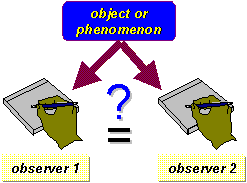Whenever you use humans as a part of your measurement procedure, you have to worry about whether the results you get are reliable or consistent. People are notorious for their inconsistency. We are easily distractible. We get tired of doing repetitive tasks. We daydream. We misinterpret.

So how do we determine whether two observers are being consistent in their observations? You probably should establish inter-rater reliability outside of the context of the measurement in your study. After all, if you use data from your study to establish reliability, and you find that reliability is low, you’re kind of stuck. Probably it’s best to do this as a side study or pilot study. And, if your study goes on for a long time, you may want to reestablish inter-rater reliability from time to time to assure that your raters aren’t changing.

There are two major ways to actually estimate inter-rater reliability. If your measurement consists of categories – the raters are checking off which category each observation falls in – you can calculate the percent of agreement between the raters. For instance, let’s say you had 100 observations that were being rated by two raters. For each observation, the rater could check one of three categories. Imagine that on 86 of the 100 observations the raters checked the same category. In this case, the percent of agreement would be 86%. OK, it’s a crude measure, but it does give an idea of how much agreement exists, and it works no matter how many categories are used for each observation.

The other major way to estimate inter-rater reliability is appropriate when the measure is a continuous one. There, all you need to do is calculate the correlation between the ratings of the two observers. For instance, they might be rating the overall level of activity in a classroom on a `1-to-7` scale. You could have them give their rating at regular time intervals (e.g., every 30 seconds). The correlation between these ratings would give you an estimate of the reliability or consistency between the raters.

You might think of this type of reliability as “calibrating” the observers. There are other things you could do to encourage reliability between observers, even if you don’t estimate it. For instance, I used to work in a psychiatric unit where every morning a nurse had to do a ten-item rating of each patient on the unit. Of course, we couldn’t count on the same nurse being present every day, so we had to find a way to assure that any of the nurses would give comparable ratings. The way we did it was to hold weekly “calibration” meetings where we would have all of the nurses ratings for several patients and discuss why they chose the specific values they did. If there were disagreements, the nurses would discuss them and attempt to come up with rules for deciding when they would give a “3” or a “4” for a rating on a specific item. Although this was not an estimate of reliability, it probably went a long way toward improving the reliability between raters.

## Test-Retest Reliability

We estimate test-retest reliability when we administer the same test to the same sample on two different occasions. This approach assumes that there is no substantial change in the construct being measured between the two occasions. The amount of time allowed between measures is critical. We know that if we measure the same thing twice that the correlation between the two observations will depend in part by how much time elapses between the two measurement occasions. The shorter the time gap, the higher the correlation; the longer the time gap, the lower the correlation. This is because the two observations are related over time – the closer in time we get the more similar the factors that contribute to error. Since this correlation is the test-retest estimate of reliability, you can obtain considerably different estimates depending on the interval.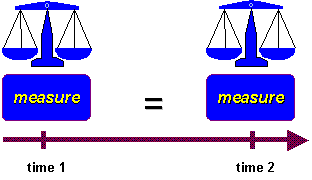## Parallel-Forms Reliability

In parallel forms reliability you first have to create two parallel forms. One way to accomplish this is to create a large set of questions that address the same construct and then randomly divide the questions into two sets. You administer both instruments to the same sample of people. The correlation between the two parallel forms is the estimate of reliability. One major problem with this approach is that you have to be able to generate lots of items that reflect the same construct. This is often no easy feat. Furthermore, this approach makes the assumption that the randomly divided halves are parallel or equivalent. Even by chance this will sometimes not be the case. The parallel forms approach is very similar to the split-half reliability described below. The major difference is that parallel forms are constructed so that the two forms can be used independent of each other and considered equivalent measures. For instance, we might be concerned about a testing threat to internal validity. If we use Form A for the pretest and Form B for the posttest, we minimize that problem. it would even be better if we randomly assign individuals to receive Form A or B on the pretest and then switch them on the posttest. With split-half reliability we have an instrument that we wish to use as a single measurement instrument and only develop randomly split halves for purposes of estimating reliability.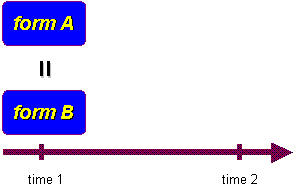## Internal Consistency Reliability

In internal consistency reliability estimation we use our single measurement instrument administered to a group of people on one occasion to estimate reliability. In effect we judge the reliability of the instrument by estimating how well the items that reflect the same construct yield similar results. We are looking at how consistent the results are for different items for the same construct within the measure. There are a wide variety of internal consistency measures that can be used.

### Average Inter-item Correlation

The average inter-item correlation uses all of the items on our instrument that are designed to measure the same construct. We first compute the correlation between each pair of items, as illustrated in the figure. For example, if we have six items we will have 15 different item pairings (i.e., 15 correlations). The average interitem correlation is simply the average or mean of all these correlations. In the example, we find an average inter-item correlation of .90 with the individual correlations ranging from .84 to .95.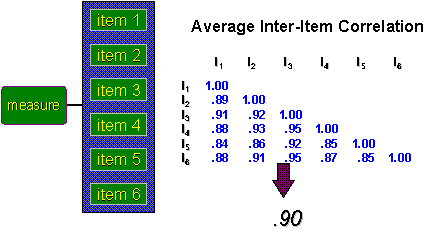### Average Itemtotal Correlation

This approach also uses the inter-item correlations. In addition, we compute a total score for the six items and use that as a seventh variable in the analysis. The figure shows the six item-to-total correlations at the bottom of the correlation matrix. They range from .82 to .88 in this sample analysis, with the average of these at .85.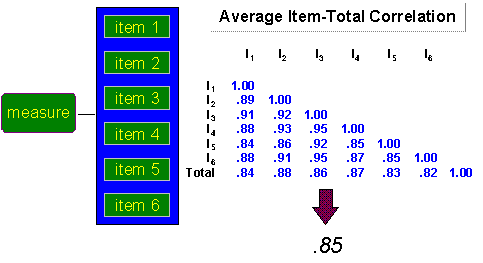### Split-Half Reliability

In split-half reliability we randomly divide all items that purport to measure the same construct into two sets. We administer the entire instrument to a sample of people and calculate the total score for each randomly divided half. the split-half reliability estimate, as shown in the figure, is simply the correlation between these two total scores. In the example it is .87.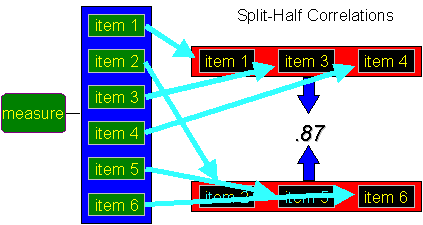### Cronbach’s Alpha (a)

Imagine that we compute one split-half reliability and then randomly divide the items into another set of split halves and recompute, and keep doing this until we have computed all possible split half estimates of reliability. Cronbach’s Alpha is mathematically equivalent to the average of all possible split-half estimates, although that’s not how we compute it. Notice that when I say we compute all possible split-half estimates, I don’t mean that each time we go an measure a new sample! That would take forever. Instead, we calculate all split-half estimates from the same sample. Because we measured all of our sample on each of the six items, all we have to do is have the computer analysis do the random subsets of items and compute the resulting correlations. The figure shows several of the split-half estimates for our six item example and lists them as SH with a subscript. Just keep in mind that although Cronbach’s Alpha is equivalent to the average of all possible split half correlations we would never actually calculate it that way. Some clever mathematician (Cronbach, I presume!) figured out a way to get the mathematical equivalent a lot more quickly.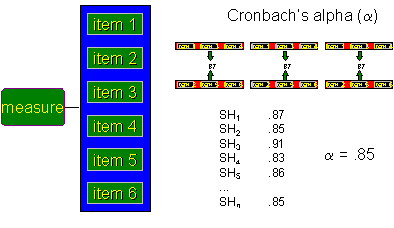## Comparison of Reliability Estimators

Each of the reliability estimators has certain advantages and disadvantages. Inter-rater reliability is one of the best ways to estimate reliability when your measure is an observation. However, it requires multiple raters or observers. As an alternative, you could look at the correlation of ratings of the same single observer repeated on two different occasions. For example, let’s say you collected videotapes of child-mother interactions and had a rater code the videos for how often the mother smiled at the child. To establish inter-rater reliability you could take a sample of videos and have two raters code them independently. To estimate test-retest reliability you could have a single rater code the same videos on two different occasions. You might use the inter-rater approach especially if you were interested in using a team of raters and you wanted to establish that they yielded consistent results. If you get a suitably high inter-rater reliability you could then justify allowing them to work independently on coding different videos. You might use the test-retest approach when you only have a single rater and don’t want to train any others. On the other hand, in some studies it is reasonable to do both to help establish the reliability of the raters or observers.

The parallel forms estimator is typically only used in situations where you intend to use the two forms as alternate measures of the same thing. Both the parallel forms and all of the internal consistency estimators have one major constraint – you have to have multiple items designed to measure the same construct. This is relatively easy to achieve in certain contexts like achievement testing (it’s easy, for instance, to construct lots of similar addition problems for a math test), but for more complex or subjective constructs this can be a real challenge. If you do have lots of items, Cronbach’s Alpha tends to be the most frequently used estimate of internal consistency.

The test-retest estimator is especially feasible in most experimental and quasi-experimental designs that use a no-treatment control group. In these designs you always have a control group that is measured on two occasions (pretest and posttest). the main problem with this approach is that you don’t have any information about reliability until you collect the posttest and, if the reliability estimate is low, you’re pretty much sunk.

Each of the reliability estimators will give a different value for reliability. In general, the test-retest and inter-rater reliability estimates will be lower in value than the parallel forms and internal consistency ones because they involve measuring at different times or with different raters. Since reliability estimates are often used in statistical analyses of quasi-experimental designs (e.g. the analysis of the nonequivalent group design), the fact that different estimates can differ considerably makes the analysis even more complex.